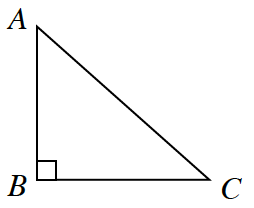### Home > INT2 > Chapter 8 > Lesson 8.2.2 > Problem8-40

8-40.

For the triangle at right, write each of the following trigonometric ratios. The first one is done for you.

Refer to the Math Notes box in Lesson 4.2.2 if you need help remembering any trigonometric ratios.1. tan $C=\frac{AB}{BC}$

1. sin $C$

1. tan $A$

1. cos $C$

$\cos{C}=\frac{BC}{AC}$

1. cos $A$

1. sin $A$

$\sin{A}=\frac{BC}{AC}$